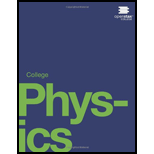# Find the gauge and absolute pressures in the balloon and peanut jar shown in Figure 11.16, assuming the manometer connected to the balloon uses water whereas the manometer connected to the jar contains mercury. Express in units of centimeters of water for the balloon and millimeters of mercury for the jar, taking h = 0.0500 m for each. Figure 11.16 An open-tube manometer has one side open to the atmosphere. (a) Fluid depth must be the same on both sides, or the pressure each side exerts at the bottom will be unequal and there will be flow from the deeper side. (b) A positive gauge pressure P g = h ρ g transmitted to one side of the manometer can support a column of fluid of height h. (c) Similarly, atmospheric pressure is greater than a negative gauge pressure P g by an amount h ρ g .The jar's rigidity prevents atmospheric pressure from being transmitted to the peanuts.### College Physics

1st Edition
Paul Peter Urone + 1 other
Publisher: OpenStax College
ISBN: 9781938168000

#### Solutions

Chapter
Section### College Physics

1st Edition
Paul Peter Urone + 1 other
Publisher: OpenStax College
ISBN: 9781938168000
Chapter 11, Problem 29PE
Textbook Problem
833 views

## Find the gauge and absolute pressures in the balloon and peanut jar shown in Figure 11.16, assuming the manometer connected to the balloon uses water whereas the manometer connected to the jar contains mercury. Express in units of centimeters of water for the balloon and millimeters of mercury for the jar, taking h = 0.0500 m for each.Figure 11.16 An open-tube manometer has one side open to the atmosphere. (a) Fluid depth must be the same on both sides, or the pressure each side exerts at the bottom will be unequal and there will be flow from the deeper side. (b) A positive gauge pressure P g = h ρ g transmitted to one side of the manometer can support a column of fluid of height h. (c) Similarly, atmospheric pressure is greater than a negative gauge pressure P g by an amount h ρ g .The jar's rigidity prevents atmospheric pressure from being transmitted to the peanuts.

To determine

The gauge and absolute pressures in the balloon and peanut jar shown in Figure, assuming the manometer connected to the balloon uses water whereas the manometer connected to the jar contains mercury? Express in units of centimeters of water for the balloon and millimeters of mercury for the jar, taking h = 0.0500 m for each.### Explanation of Solution

Given info:

h=0.0500m

Formula used:

Pressure,

P=hρg , h is the height, ρ is the density and g is acceleration due to gravity.

Absolute pressure,

Pabs=Pg+Patm

Calculation:

Balloon

Pabs=Pg+PatmPg=0.0500 m of H2O     = 5 cm of H2OPabs=(0.0500+1013251000×9.81)=10.38 m of H2O      = 1.038×103cm of H2O

Peanut jar

Pabs=Pg+PatmPg=0

### Still sussing out bartleby?

Check out a sample textbook solution.

See a sample solution

#### The Solution to Your Study Problems

Bartleby provides explanations to thousands of textbook problems written by our experts, many with advanced degrees!

Get Started

Find more solutions based on key concepts
The theory that vitamin C prevents or cures colds is well supported by research. T F

Nutrition: Concepts and Controversies - Standalone book (MindTap Course List)

What are the base-pairing rules for DNA? a. AG, TC b. AT, GC c. AC, TG d. AA, GG, CC, TT

Biology: The Unity and Diversity of Life (MindTap Course List)

What chemical condition is responsible for jaundice?

General, Organic, and Biological Chemistry

The device shown in Figure CQ22.7, called a thermoelectric converter, uses a series of semiconductor cells to t...

Physics for Scientists and Engineers, Technology Update (No access codes included)

Why is plastic so dangerous to marine organisms?

Oceanography: An Invitation To Marine Science, Loose-leaf Versin